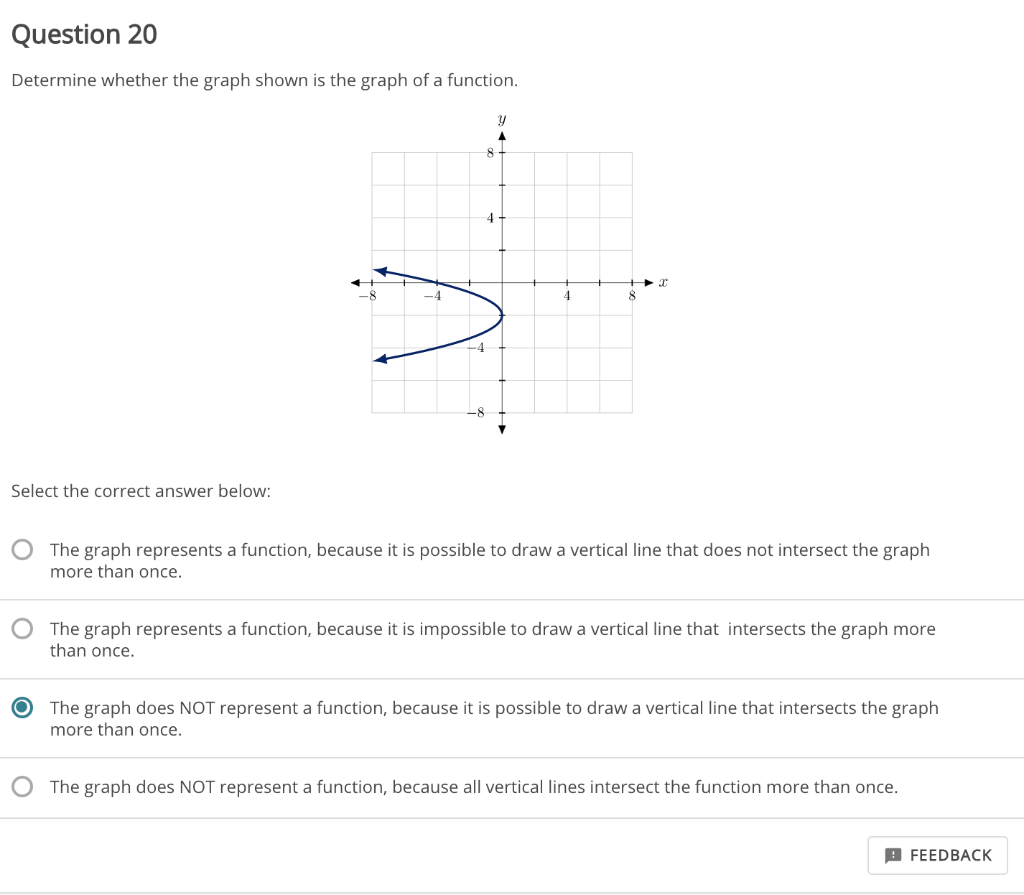# Which graph represents a functionQuestion 20 Determine whether the graph shown is the graph of a function. -8 Select the correct answer below: O The graph represents a function, because it is possible to draw a vertical line that does not intersect the graph more than once. The graph represents a function, because it is impossible to draw a vertical line that intersects the graph more than once. The graph does NOT represent a function, because it is possible to draw a vertical line that intersects the graph more than once. O The graph does NOT represent a function, because all vertical lines intersect the function more than once. FEEDBACK

Solved
Option c
Is correct, the graph does not pass the vertical line test.
Since vertical line cuts the graph more than once.